# Spherical Lens

Spherical lenses are optical systems capable of promoting the refraction of visible light. They are formed by homogeneous and transparent optical means, which can also be called spherical diopters. They are divided into concave and convex lenses , which are, respectively, wide-edged lenses and thin-edged lenses.

The refractive index and geometry of spherical lenses change the direction of propagation of light rays. In this way, they are able to produce both virtual and real images. Furthermore, the ability of a lens to deflect light rays is called vergence or diopter , a property popularly known as the “degree” of the lens.

## Properties of spherical lenses

The properties of spherical lenses may vary according to the medium in which they are found. When inserted in a medium whose refractive index is lower than that of the lens itself, convex lenses converge light , while concave lenses diverge light , as shown in the following figure:

In the middle of each lens you can see lines with arrows in and out. These symbols are used to represent thin-edged (convex) and wide-edged (concave) lenses. In total, there are three concave lens shapes and three convex lens shapes. Check your nomenclature:

The shape of the lenses interferes with their ability to deflect the path of light. This lens behavior is explained by Halley’s equation , which will be shown later in this text.

What are spherical lenses for?

Spherical lenses are used to correct vision problems such as myopia, astigmatism, hyperopia, etc. In addition, they are indispensable for the operation of various optical instruments , such as microscopes, telescopes, telescopes, binoculars, magnifying glasses, among others.

## Geometric elements of spherical lenses

Every spherical lens, whether concave or convex, has geometric elements in common, namely:

• optical center (O);
• main focus object and main focus image (F and F’);
• anti-principal object focus and anti-principal image focus, also known as centers of curvature in the case of spherical mirrors (A and A’ or C and C’).

The following figure shows where the geometric elements of any spherical lens are located , observe:

Based on the image above, we say that the distance between the optical center of the lens and the main focus of the object is called the focal length (f); likewise, the distance between the optical center and the antiprincipal object focus is called the radius of curvature.

In the figure above, it is also possible to observe a horizontal line that separates the lens into two parts. This line is called the axis of symmetry and it is from this that the Gauss frame of reference is constructed, used to define the sign convention of spherical lenses. According to the Gaussian frame of reference:

• any point or element that is located above the symmetry axis has a positive sign;
• any point or element located below the axis of symmetry has a negative sign;
• any point or element located to the right of the optical center of the lens has a positive sign;
• any point or element located to the left of the optical center of the lens has a negative sign.

The following figure will help us understand the sign convention according to the Gaussian frame of reference. Check out:

In addition to the conventions arbitrated by the Gaussian frame of reference, there is some useful information that can be taken into account when solving exercises. Both the main focus and anti-primary focus of diverging lenses such as concave lenses are negative. Also, when the image size (i) has the minus sign, this indicates that it is inverted. Finally, if we find that the position of an image (p’) is positive, this indicates that this image is real; otherwise, if the position of the image were negative, that image would be virtual.

## remarkable rays

The remarkable rays are necessary for us to understand how image formation works in spherical lenses . These are light rays that are always refracted over certain geometric elements specific to spherical lenses. Furthermore, it is necessary to remember one of the principles of geometric optics: the reversibility of light rays. When we draw a ray of light going from one point to another, this ray can either be on the “going” path or the “back” path.

According to the image above, every ray of light that:

• I – incident parallel to the symmetry axis is refracted over the main focus (F); / falls on the main focus is refracted parallel to the axis of symmetry.
• II – focuses on the object’s anti-main focus (A) is refracted onto the image’s main focus (A’); / focuses on the image anti-primary focus (A’) is refracted onto the object’s anti-primary focus (A).
• III- incident on the optical center is refracted without suffering any lateral deviation.

## Image formation in spherical lenses

The formation of images in spherical lenses requires us to trace the remarkable light rays that are refracted through the lens. The image is always formed at the point where two refracted light rays intersect . When the image is formed on the same side as the object, this image is qualified as virtual; when the image is formed on the side of the lens opposite the object, it will be real.

Check here what are the characteristics of real and virtual images:

• Real image: it can be projected, it is inverted and formed by the crossing of at least two rays of light.
• Virtual image: every virtual image cannot be projected, it is straight and formed by the crossing of extensions of light rays.

In addition, the formation of images in spherical lenses depends on the position of the object in relation to the optical center of the lens and also on the shape of the lens, which can be either concave or convex. For concave lenses, there is only one case of image formation: all images formed by concave lenses are virtual, formed by the crossing of extensions of light rays and always smaller than the object of the image.

pe p’ – object and image positions

o – object height

i – image height

In the case of thin-edged lenses, imaging is more complex and involves a total of five cases. Let’s look at them one by one, starting with the situation where an object is far from the lens and its anti-principal focus. In this case, the image formed is inverted, real, formed by the intersection of light rays and smaller than the object.

When the object is brought close to the lens and placed exactly over the anti-principal focus, the image formed is real, inverted, and the same size as the object. Watch:

Bringing the object a little closer to the lens will place it between the anti-main and main focus, respectively. In this case, the image conjugated by the lens will be real, inverted and increasing, according to the approximation.

When the object is placed at a distance from the optical center of the convex lens that coincides with its focal length, that is, when the object is placed on top of the main focus, there is no image formation, since the rays of light refracted by the lens are parallel and therefore do not intersect. In this case, we say that the image is improper and that it is formed at infinity.

The last case is where the object is placed close to the convex lens, between the main focus and the optical center. In this case, the refracted light rays are divergent, so the image formed is virtual, formed by the crossing of extensions of light rays and larger than the object.

## Vergence, diopter and juxtaposition of spherical lenses

Vergence is a measure of a lens’ ability to deflect the path of light. It is symbolized by the letter C, and its unit of measurement is the diopter (di), which is equivalent to -1 . A positive vergence indicates that the lens is converging, while a negative vergence indicates that it is diverging. The vergence of a lens can be easily calculated if we know the focal length of the lens.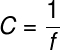## Juxtaposition of spherical lenses

Spherical lens juxtaposition is the process of combining different lenses to obtain different vergence configurations. Additionally, this process is used to reduce an effect called chromatic aberration, but it can also be used to enhance the effect achieved by a single lens.

Chromatic aberration happens when light travels a long way inside a lens, causing the light refracted by the lens to scatter , thus separating its components, just as light passes through a prism .

The figure below shows the juxtaposition of two spherical lenses with vergence C 1 and C 2 . The equivalent vergence of this combination is equal to the sum of the individual vergences.

## Spherical lens formulas

The main formulas used to solve exercises on spherical lenses use the variables:

• f – focal length;
• pep ‘ – object and image position;
• o – object size;
• i – image size;
• A – transverse linear increase or enlargement;
• R – radius of curvature;
• C – vergence.

The most basic formula for spherical lenses is known as linear transverse magnification or magnification. Lens magnification can be calculated using three different formulas, which can be combined with each other. Check out: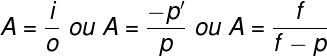In addition to the above formula, there is also a formula known as the conjugate point equation or Gauss equation. Look: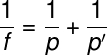The following formula is used to calculate the vergence or degree of spherical lenses:Finally, the following formula, known as Halley’s equation or lens maker’s equation, is used to determine the vergence of a spherical lens based on the refractive index of the lens material and the medium the lens is in. (air, for example), in addition to the radii of curvature of the outer and inner faces of the lens. Check out: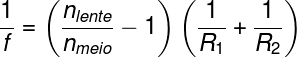## Solved exercises on spherical lenses

Question 1 – A 10 cm object is placed 15 cm from a convex spherical lens with a focal length of 5 cm. Determine:

a) the size of the image of the object;

b) the position of the image;

c) the characteristics of the image.

Resolution:

a) To find out the size of the image, we can use the formula for linear transverse increase. Watch: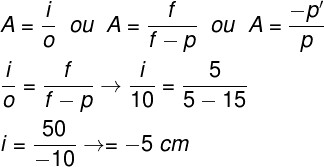The calculation made indicates that the image will have a size of 5 cm. Also, the negative sign obtained in the result indicates that the image is turned upside down.

b) The position of the image can be obtained either through the equation of the conjugate points or through one of the formulas of the transversal linear increase, as we did in question 1.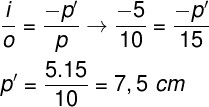The result obtained shows that the image is located 7.5 cm from the optical center of the lens. Since the signal found was positive, we know that the image produced is real.

c) The image obtained is real, since the size of this image has a negative sign, and it is also reduced, that is, smaller than the object.

Question 2) Determine the vergence of a concave spherical lens whose focal length is 10 cm.

Resolution :

Let’s calculate what the vergence of the lens is. Therefore, it is necessary to remember that, in the case of a concave lens, its focus is negative. Also, this focus must be in meters in order to calculate the vergence correctly, so we use f = -0.1 m and do the following calculation: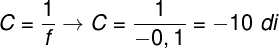The result obtained shows that this lens has a vergence equal to -10 di.

Check Also
Close